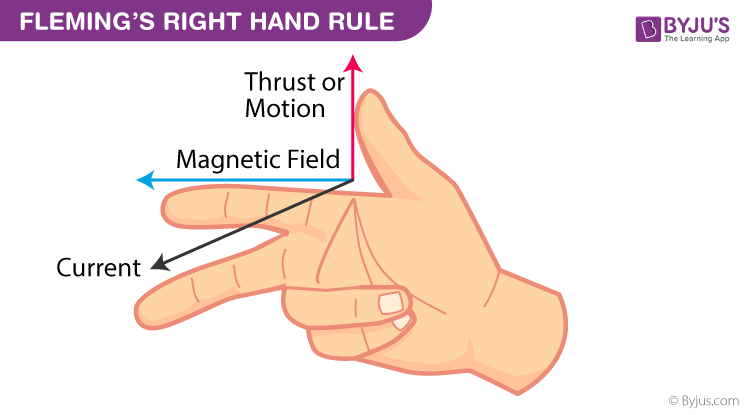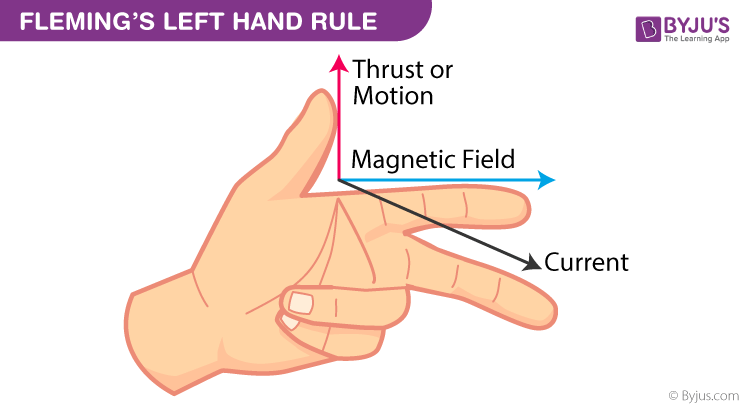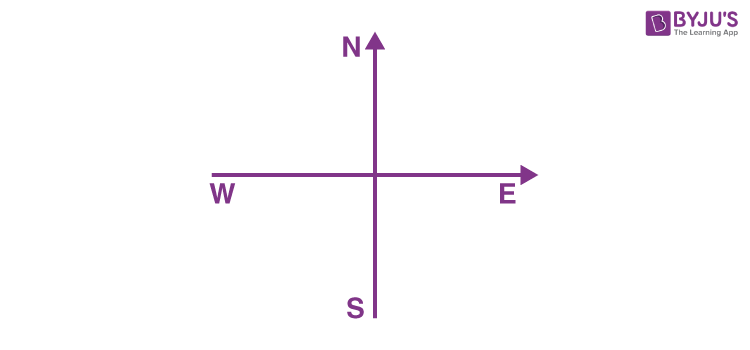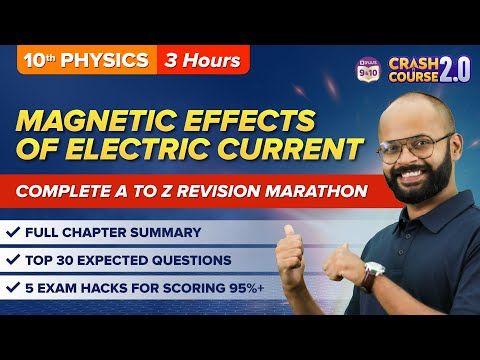# Fleming’s Left-Hand Rule and Fleming’s Right-Hand Rule

Fleming’s Left-Hand Rule and Fleming’s Right-Hand Rule are essential rules applicable in magnetism and electromagnetism. John Ambrose Fleming developed them in the late 19th century as a simple way of working out the direction of electric current in an electric generator or the direction of motion in an electric motor. It is important to note that these rules do not determine the magnitude; instead show only the direction of the three parameters (magnetic field, current, force) when the direction of the other two parameters is known.

## What Is Fleming’s Right-Hand Rule?

According to Faraday’s law of electromagnetic induction, when a conductor moves through a magnetic field, an electric current is induced in it. Fleming’s right-hand rule is used to determine the direction of the induced current.Fleming’s Right Hand Rule states that if we arrange our thumb, forefinger and middle finger of the right-hand perpendicular to each other, then the thumb points towards the direction of the motion of the conductor relative to the magnetic field, the forefinger points towards the direction of the magnetic field and the middle finger points towards the direction of the induced current.

## What is Fleming’s Left-Hand Rule?

When a current-carrying conductor is placed in an external magnetic field, the conductor experiences a force perpendicular to both the field and the current flow’s direction. Fleming’s left-hand rule is used to find the direction of the force acting on the current carrying conductor placed in a magnetic field.Fleming’s Left Hand Rule states that if we arrange our thumb, forefinger and middle finger of the left-hand perpendicular to each other, then the thumb points towards the direction of the force experienced by the conductor, the forefinger points towards the direction of the magnetic field and the middle finger points towards the direction of the electric current.

## Tips to remember Fleming’s Left Hand & Right-Hand Rule## Difference Between Fleming’s Left-Hand and Right-Hand Rule

 Fleming’s Left-Hand Rule Fleming’s Right-Hand Rule It is used for electric motors It is used for electric generators The purpose of this rule is used for finding the direction of the magnetic force acting in an electric motor. The purpose of this rule is used for finding the direction of the induced current in an electric generator. The middle finger represents the direction of the current The middle finger represents the direction of the induced current

We can observe that the left-hand rule is applicable to Motors and the right-hand rule to Generators. Fleming’s left-hand rule and Fleming’s right-hand rule are a pair of visual mnemonics (mnemonics are learning techniques or memory aids, such as an abbreviation, rhyme or mental image that helps to remember something). In practice, these rules are never used except as a convenient trick to determine the direction of the resultant – either current or thrust. What gives the magnitude of force along this direction determined by these rules is the Lorentz’ Force.

In this episode of Class 10 One-Shot Series, we take up CBSE Class 10 Science Chapter 13 – Magnetic Effects of Electric Current. We help you understand all concepts related to the Magnetic Field, Magnetic Field Due to a Current-Carrying Conductor, Oersted’s experiment, Right-hand thumb rule, Fleming’s left-hand rule, Faraday’s experiment, Fleming’s right-hand rule, and Electromagnetic induction.### Fleming’s Left-Hand Rule Examples

Q1. Determine the direction of the force acting on the proton if the proton moves towards the east by entering a uniform magnetic field in the downward direction.

Using Fleming’s left-hand rule, we can determine the direction of force acting on the proton.It is given that the proton is moving towards the east, and therefore, the motion of the current is towards the east. The direction of force is towards the north as the magnetic field is acting downwards. Consequently, we can say that the direction of the force acting is towards the north.

Q2. Find the direction of the magnetic field if an electron is moving vertically upwards and gets deflected towards the south due to a uniform magnetic field.

Using Fleming’s left-hand rule, we can determine the direction of the magnetic field acting on the electron.We know that the charge on an electron is negative. When the electron moves upward, the direction of the current is in the opposite direction; that is, the direction of the current is downwards. It is given that the force acting on the electron is in the south direction. Therefore, the direction of the magnetic field is towards the east.

## Moving Charges and Magnetism Lecture in Hindi## Frequently Asked Questions – FAQs

Q1

### State Fleming’s right hand rule.

Fleming’s Right Hand Rule states that if we arrange our thumb, forefinger and middle finger of the right-hand perpendicular to each other, then the thumb points towards the direction of the magnetic force, the forefinger points towards the direction of the magnetic field and the middle finger points towards the direction of the current.
Q2

### State Fleming’s left-hand rule.

Fleming’s Left Hand Rule states that if we arrange our thumb, forefinger and middle finger of the left hand perpendicular to each other, then the thumb points towards the direction of the magnetic force, the forefinger points towards the direction of the magnetic field and the middle finger points towards the direction of the current.
Q3

### Which rule is used in electric motors?

Fleming’s Left-Hand rule is used in electric motors.
Q4

### What is the purpose of Fleming’s right hand rule?

The purpose of Fleming’s right hand rule is to find the direction of induced current when a conductor moves in a magnetic field.
Q5

### Which rule is used for electric generators?

Fleming’s right-hand rule is used for electric generators.

## The below videos help to revise the chapter Magnetic Effects of Electric Current Class 10